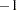The SCORE Procedure
 PROC SCORE Statement

PROC SCORE DATA= SAS-data-set <options> ;

You can specify the following options in the PROC SCORE statement.

DATA=SAS-data-set

names the input SAS data set containing the raw data to score. This option is required.

NOSTD

suppresses centering and scaling of the raw data. Ordinarily, if PROC SCORE finds _TYPE_=‘MEAN’, _TYPE_= ‘USCORE’, _TYPE_=‘USTD’, or _TYPE_=‘STD’ observations in the SCORE= data set, the procedure uses these to standardize the raw data before scoring.

OUT=SAS-data-set

specifies the name of the SAS data set created by PROC SCORE. If you want to create a permanent SAS data set, you must specify a two-level name. See SAS Language Reference: Concepts for more information about permanent SAS data sets. If the OUT= option is omitted, PROC SCORE still creates an output data set and automatically names it according to the DATAconvention, as if you omitted a data set name in a DATA statement.

PREDICT

specifies that PROC SCORE should treat coefficients ofin the SCORE= data set as 0. In regression applications, the dependent variable is coded with a coefficient of. Applied directly to regression results, PROC SCORE produces negative residuals (see the description of the RESIDUAL option, which follows); the PREDICT option produces predicted values instead.

RESIDUAL

reverses the sign of each score. Applied directly to regression results, PROC SCORE produces negative residuals (PREDICTACTUAL); the RESIDUAL option produces positive residuals (ACTUALPREDICT) instead.

SCORE=SAS-data-set

names the data set containing the scoring coefficients. If you omit the SCORE= option, the most recently created SAS data set is used. This data set must have two special variables: _TYPE_ and either _NAME_ or _MODEL_.

TYPE=name or ‘string’

specifies the observations in the SCORE= data set that contain scoring coefficients. The TYPE= procedure option is unrelated to the data set option that has the same name. PROC SCORE examines the values of the special variable _TYPE_ in the SCORE= data set. When the value of _TYPE_ matches TYPE=name, the observation in the SCORE= data set is used to score the raw data in the DATA= data set.

If you omit the TYPE= option, scoring coefficients are read from observations with either _TYPE_=’SCORE’ or _TYPE_=’USCORE’. Because the default for PROC SCORE is TYPE=SCORE, you need not specify the TYPE= option for factor scoring or for computing scores from OUTSTAT= data sets from the CANCORR, CANDISC, PRINCOMP, or VARCLUS procedure. When you use regression coefficients from PROC REG, specify TYPE=PARMS.

The maximum length of the argument specified in the TYPE= option depends on the length defined by the VALIDVARNAME= SAS system option. For additional information, see SAS Language Reference: Dictionary.

Note that the TYPE= option setting is not case sensitive. For example, the two option settings TYPE=’MyScore’ and TYPE=’myscore’ are equivalent.Previous Page | Next Page | Top of Page